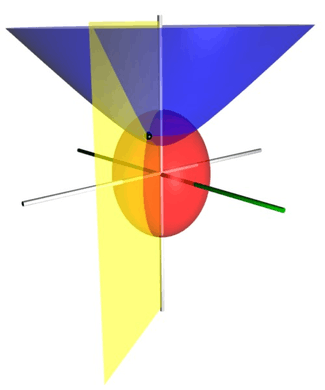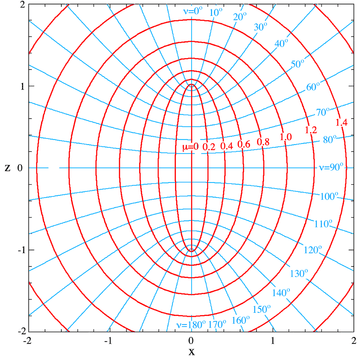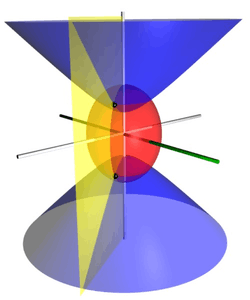# Prolate spheroidal coordinatesThe three coordinate surfaces of prolate spheroidal coordinates. The red prolate spheroid (stretched sphere) corresponds to μ=1, and the blue two-sheet hyperboloid corresponds to ν=45°. The yellow half-plane corresponds to φ=-60°, which is measured relative to the x-axis (highlighted in green). The black sphere represents the intersection point of the three surfaces, which has Cartesian coordinates of roughly (0.831, -1.439, 2.182).

Prolate spheroidal coordinates are a three-dimensional orthogonal coordinate system that results from rotating the two-dimensional elliptic coordinate system about the focal axis of the ellipse, i.e., the symmetry axis on which the foci are located. Rotation about the other axis produces oblate spheroidal coordinates. Prolate spheroidal coordinates can also be considered as a limiting case of ellipsoidal coordinates in which the two smallest principal axes are equal in length.

Prolate spheroidal coordinates can be used to solve various partial differential equations in which the boundary conditions match its symmetry and shape, such as solving for a field produced by two centers, which are taken as the foci on the z-axis. One example is solving for the wavefunction of an electron moving in the electromagnetic field of two positively charged nuclei, as in the hydrogen molecular ion, H2+. Another example is solving for the electric field generated by two small electrode tips. Other limiting cases include areas generated by a line segment (μ=0) or a line with a missing segment (ν=0).

## DefinitionProlate spheroidal coordinates μ and ν for a=1. The lines of equal values of μ and ν are shown on the x-z plane, i.e. for φ=0. The surfaces of constant μ and ν are obtained by rotation about the z axis, so that the diagram is valid for any plane containing the z axis: i.e. for any φ.

The most common definition of prolate spheroidal coordinatesiswhereis a nonnegative real number and. The azimuthal anglebelongs to the interval.

The trigonometric identityshows that surfaces of constantform prolate spheroids, since they are ellipses rotated about the axis joining their foci. Similarly, the hyperbolic trigonometric identityshows that surfaces of constantform hyperboloids of revolution.

The distances from the foci located atare## Scale factors

The scale factors for the elliptic coordinatesare equalwhereas the azimuthal scale factor equalsConsequently, an infinitesimal volume element equalsand the Laplacian can be writtenOther differential operators such asandcan be expressed in the coordinatesby substituting the scale factors into the general formulae found in orthogonal coordinates.

## Alternative definitionIn principle, a definition of prolate spheroidal coordinates could be degenerate. In other words, a single set of coordinates might correspond to two points in Cartesian coordinates; this is illustrated here with two black spheres, one on each sheet of the hyperboloid and located at (x, y, ±z). However, neither of the definitions presented here are degenerate.

An alternative and geometrically intuitive set of prolate spheroidal coordinatesare sometimes used, whereand. Hence, the curves of constantare prolate spheroids, whereas the curves of constantare hyperboloids of revolution. The coordinatebelongs to the interval [-1, 1], whereas thecoordinate must be greater than or equal to one.

The coordinatesandhave a simple relation to the distances to the fociand. For any point in the plane, the sumof its distances to the foci equals, whereas their differenceequals. Thus, the distance tois, whereas the distance tois. (Recall thatandare located atand, respectively.) This gives the following expressions for,, and:Unlike the analogous oblate spheroidal coordinates, the prolate spheroid coordinates (σ, τ, φ) are not degenerate; in other words, there is a unique, reversible correspondence between them and the Cartesian coordinates## Alternative scale factors

The scale factors for the alternative elliptic coordinatesarewhile the azimuthal scale factor is nowHence, the infinitesimal volume element becomesand the Laplacian equalsOther differential operators such asandcan be expressed in the coordinatesby substituting the scale factors into the general formulae found in orthogonal coordinates.

As is the case with spherical coordinates, Laplace's equation may be solved by the method of separation of variables to yield solutions in the form of prolate spheroidal harmonics, which are convenient to use when boundary conditions are defined on a surface with a constant prolate spheroidal coordinate (See Smythe, 1968).

## Bibliography

### No angles convention

• Morse PM, Feshbach H (1953). Methods of Theoretical Physics, Part I. New York: McGraw-Hill. p. 661. Uses ξ1 = a cosh μ, ξ2 = sin ν, and ξ3 = cos φ.
• Zwillinger D (1992). Handbook of Integration. Boston, MA: Jones and Bartlett. p. 114. ISBN 0-86720-293-9. Same as Morse & Feshbach (1953), substituting uk for ξk.
• Smythe, WR (1968). Static and Dynamic Electricity (3rd ed.). New York: McGraw-Hill.
• Sauer R, Szabó I (1967). Mathematische Hilfsmittel des Ingenieurs. New York: Springer Verlag. p. 97. LCCN 67025285. Uses coordinates ξ = cosh μ, η = sin ν, and φ.

### Angle convention

• Korn GA, Korn TM (1961). Mathematical Handbook for Scientists and Engineers. New York: McGraw-Hill. p. 177. LCCN 59014456. Korn and Korn use the (μ, ν, φ) coordinates, but also introduce the degenerate (σ, τ, φ) coordinates.
• Margenau H, Murphy GM (1956). The Mathematics of Physics and Chemistry. New York: D. van Nostrand. pp. 180182. LCCN 55010911. Similar to Korn and Korn (1961), but uses colatitude θ = 90° - ν instead of latitude ν.
• Moon PH, Spencer DE (1988). "Prolate Spheroidal Coordinates (η, θ, ψ)". Field Theory Handbook, Including Coordinate Systems, Differential Equations, and Their Solutions (corrected 2nd ed., 3rd print ed.). New York: Springer Verlag. pp. 2830 (Table 1.06). ISBN 0-387-02732-7. Moon and Spencer use the colatitude convention θ = 90° - ν, and rename φ as ψ.

### Unusual convention

• Landau LD, Lifshitz EM, Pitaevskii LP (1984). Electrodynamics of Continuous Media (Volume 8 of the Course of Theoretical Physics) (2nd ed.). New York: Pergamon Press. pp. 1929. ISBN 978-0-7506-2634-7. Treats the prolate spheroidal coordinates as a limiting case of the general ellipsoidal coordinates. Uses (ξ, η, ζ) coordinates that have the units of distance squared.